## MaplePrimes Questions

Search Questions:

## double negative...

Why can't I do double negatives and why is it so difficult to do simple mathematics with negatives?

(a - b)

want to simply rewrite this by substitution for a simple quick calculation, repalce a and b with their values, e.g.,

-3 - -5

Maple complains!

4*-10

maple complains!

This makes it impossible to not have to rewrite simple expressions just to get maple to work.

Surely there is a untary negative sign that I can use that will please maple?

## Can we improve on the accuracy of this fractal gra...

I am trying to reproduce this fractal graphic by Daniel Geisler, using maple 18:

http://tetration.org/Tetration/index.html

I suspect the images were produced by fractint - with a floating point hardware accelerator, but I am not sure. In any case, they were produced by a numerical program, not a symbolic one and probably with 16-18 digits of accuracy.

The Maple code I use makes for a valiant effort, but is nowhere close in accuracy to the two images above. Here's the Maple 18 code:

tetrationFractal18.mw

Several questions here:

1) Geisler's image colors according to period (right graph). Period 1 is red. 2 is yellow (although not all yellow regions are period 2) 3 is green, 4 is cyan, 5 is blue, etc.

I have some period checking code in my doc, which stores the last 20 iterates of the maxIter iterations of the orbit in orb[n]  then extracts a period p, based on some backwards comparisons.

I am only interested in graphing max period up to N=20, hence the orb[1..N] array, stores only the last N elements of the iterates. This is relatively fast, but extracting the period (below) from the orb array, puts an additional strain on the overall calculations, probably of the order of O((N+p)*n) for each n in the iteration, where p is the period returned. That's why I avoid storing in orb unless the global bound "bail" has not been exceeded.

The period finding algorithm works. It rerurns p correctly, and that's the value the graphic proc returns for plot3d. The problem is that the range of values is small (p\in[1..N]) and therefore the palette in plot3d is poor. I.e., it doesn't differentiate very much between colors of different period regions. How can I spread out the color pallete with plot3d with such results? ideally, I'd like to assign colors roughly from red to violet, with period 1 red, 2 orange, 3 green, 4 blue, 5 purple, etc.

I tried returning log(p) and this spreads the zhue some, but not enough (see attached document). Further, I'd like to assign black to points which escape the calculations, such as when the bail bound is exceeded in my iteration loop. By default, these show up as "purple", which is the same as the color for period 1 with the latest implementation. How can I except these escape points from the palette, i.e. what does the fractal proc have to return to color these black? Note that for these points, orb[n] defaults to 0, so the palette colors this close to p=1 region (these are the black "hairs"/threads of the Cantor Bouquets in Geisler's second image. That's the first question.

Second question is, why are there "white" regions interlaced under this scheme? is the calculation failing at these points because of low resolution? (epsilon~0.01 in this doc). (If points fail "bound"=1e10 then the orbit is orb[1..N]=0, so p=1 and zhue=1?) White seems to decrease as I increase epsilon and maxIter, but i can't test further, because the calculation limit is already prohibitive for this version of Maple. The graph with a plot3d grid [400,400] and maxIter=100 and epsilon=0.01, takes around 25 minutes. Testing anything finer than than, is time uneasonable.

Would increasing Digits help with any of the above?

Does anyone have any ideas on how to improve the code performance for this graph in maple 18, as per the above problems?.

Many thanks.

## how to guess 1 or 2 when rsolve is wrong at some n...

second has rsolve solution

compare original sequence

accsum(ListTools[Reverse]([seq(patterngroup[k], k=(mm)..(mm+lengthofsequence))])),

with rsolve solution , it start from third number wrong, some number are wrong but correct at next number,

but i just want to guess next number is 1 or 2

[seq(subs(k=kk, sol), kk=0..(lengthofsequence+1))],

with(SumTools):
with(gfun):
accsum := proc(xx)
local result,mm:
result := []:
for mm from 1 to nops(xx) do
temp := Summation(xx[k], k = 1 .. mm):
result := [op(result), temp]:
od:
return result:
end proc:

patterngroup := [2, 1, 1, 1, 2, 2, 1, 1, 2, 2, 2, 1]: # left is latest, 2,1,1..
lengthofsequence := 5:
for mm from 1 to 12 do
if guessgf(accsum(Reverse([seq(patterngroup[k], k=(mm)..(mm+lengthofsequence))])), x) = FAIL then
print(patterngroup[mm]):
else
sol := rsolve(listtorec(accsum(ListTools[Reverse]([seq(patterngroup[k], k=(mm)..(mm+lengthofsequence))])), u(n)), u(k)):
acxxc := accsum(ListTools[Reverse]([seq(patterngroup[k], k=(mm)..(mm+lengthofsequence))])):
olist := [seq(patterngroup[k], k=(mm)..(mm+5))]:
#start from 0
print(patterngroup[mm], olist, accsum(ListTools[Reverse]([seq(patterngroup[k], k=(mm)..(mm+lengthofsequence))])), [seq(subs(k=kk, sol), kk=0..(lengthofsequence+1))], sol, subs(k=(lengthofsequence+1), sol), subs(k=(lengthofsequence+1), sol)-acxxc[nops(acxxc)]):
end if:
od:

## Kind help to enumerate permutation with repetion t...

Below is python code found in the internet kind help to convert to maple but only thing instead on the input to be string I want it to be a set of integers or array of integers

Kind help

# Python program to print all permutations with repetition

# of characters



def toString(List):

    return ''.join(List)



# The main function that recursively prints all repeated

# permutations of the given string. It uses data[] to store

# all permutations one by one

def allLexicographicRecur (string, data, last, index):

    length = len(string)



    # One by one fix all characters at the given index and

    # recur for the subsequent indexes

    for i in xrange(length):



        # Fix the ith character at index and if this is not

        # the last index then recursively call for higher

        # indexes

        data[index] = string[i]



        # If this is the last index then print the string

        # stored in data[]

        if index==last:

            print toString(data)

        else:

            allLexicographicRecur(string, data, last, index+1)



# This function sorts input string, allocate memory for data

# (needed for allLexicographicRecur()) and calls

# allLexicographicRecur() for printing all permutations

def allLexicographic(string):

    length = len(string)



    # Create a temp array that will be used by

    # allLexicographicRecur()

    data = [""] * (length+1)



    # Sort the input string so that we get all output strings in

    # lexicographically sorted order

    string = sorted(string)



    # Now print all permutaions

    allLexicographicRecur(string, data, length-1, 0)



# Driver program to test the above functions

string = "ABC"

print "All permutations with repetition of " + string + " are:"

allLexicographic(string)

## Positionvector curve...

I studiy a classroom  with 3 examples of a positionvector(radiusvector).
The last one a positionvector curve defined by a procedure  in table 3 is not yet working

• see enclosed worksheet : animate(F, [z], z = 0 .. 5, frames = 101) is not working yet

## Substitute variables into vectors with VectorCalcu...

Hello,

I'm sure this is a dumb question, can someone remind my why this doesn't work and how to get around it?

 >>>(1)
 >(2)
 >(3)
 >## Solving an optimal control problem...

Hello all

I wanna solve an optimal control problem and I have searched the Internet but I could not find any tutorial or video course on how to solve it with the Pontryagin maximum principle method. It is my first time that I want to use MAPLE for solving an optimal control problem and I would be thankful if someone can help me.

$$\max \int_{0}^{1} x_{2} [u(t)-u(t)^2] dt$$

$$\dot{x}_{0} = -(1-u(t)) x_{0}(t)+2 x_{1}(t)$$

$$\dot{x}_{1}(t) = (1-u_{t}) x_{0}(t) +2 x_{2}(t) -[3-u(t)]x_{1}(t)$$

$$\dot{x}_{2}(t) = (1-u(t))x_{1}(t) -2 x_{2}(t)$$

$$0 \le u_{t} \le \frac{1+t^2}{1+t}$$

Thanks

## Enforce non-trivial solutions when using dsolve...

Hello Everyone,

Firstly thank you for your help everyone with answering my other question yesterday, it really helped.
Today I present with yet another issue, which is dealing with solving differential equations using the dsolve-command.

I have written a script which is defining the differential equations, (some numbers), and the constraints. However when I let it solve using dsolve, I only get a very very trivial answer, meaning f(x) = 0.
of course this is a valid answer, but not one I can work with...
(Can I maybe give an additional information, e.g. the function type it is supposed to do this task? In the documentation there is information on setting it up as a series, but what about exponential equations?)

I have uploaded to script (very short), maybe someone knows where I went wrong?
Also, I am assuming that for solving a DE which involves a fourth order derivative I need exactly four boundary conditions, which I provided.
Things are also getting really wonky when I set Nxy to something non-zero... Then I get a solutions which involves a mysterious Z which never happened before and again all four C_x, which I assume resemble missing boundary conditions, reappear.

Any help would be fantastic! :)

Best Regards,

Lennart(1)## Given a lagrangian in general relativity , how ca...

Given a lagrangian  in general relativity , how can I calculate equation of motion using euler lagrangian equation in maple software ?

the lagrangian is

L = 1/2 g[mu,nu] diff(x[~mu], lambda) diff(x[~nu],lambda)

here lambda is the affine parameter.  and the metric is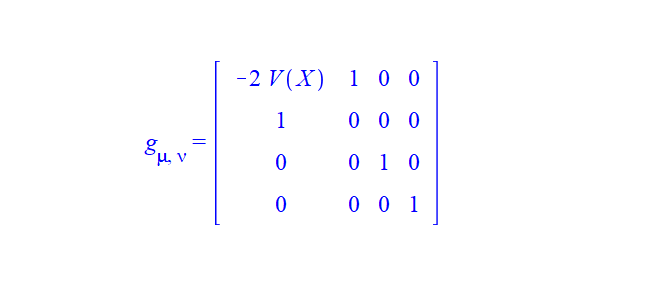metric coordinate = (t,u,x,y)

## How to find all the free variables from LinearAlge...

This is the context: I call LinearAlgebra:-LinearSolve to solve system of linear equations. If there are infinite solutions, then Maple return the solution that has free variables, calling them using  _t[n] where can change each time and be different. It can also be _t0[n] and _t1[n] etc.. but these all are indexed variables.

I want to obtain a list of all these free variables in the resulting solution vector, so that later I can assign them some values.

But I do not know what can be. And how many of them there are. There could be _t and _t for example in the same solution vector.

Here is what I do now, where I just check for indexed type. This seems to work, since only _t[n] should be in the resulting solution if any.

restart;
A:=Matrix([[0,1,-1],[-1,-2,2],[-1,-2,2]]):
v:=Vector([-1,1,1]):
sol := LinearAlgebra:-LinearSolve(A,v);

map(x->select(type,x,'indexed'),convert(sol,set))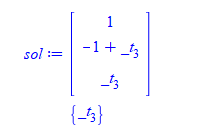I do not know how to tell it to look for all something that starts with  _t and also indexed type.

Is there a better way to obtain list of all _t[n] that LinearAlgebra:-LinearSolve could return? Will LinearAlgebra always return the free variables as indexed variables so I am sure the above will always work?  Do you see a problem with the above solution?

## how to convert a list or a set to expression seque...

It is annoying that some functions in Maple wants input like (a,b,c,.....) which represents numbers, and I am not able to find how to use such a function, because the list of numbers I have are in a list.

For example, ilcm and igcd.  This is not a good design. The input should have been a list or set, or vector, etc....

I wanted to find least common multiplier of a list of numbers. These numbers are allready in a list, since this is a result of a computation done earlier. Now I want to find ilcm of them.

How to use ilcm in this case? How to unpack them to make ilcm happy? In Mathematica there a special function to do this, called Sequence, which takes a list and unpack it to call function.

But I am not able to find one in Maple. There is no convert(list,exprseq).

Here is a MWE

v:=[2,4,7];
ilcm( v ); #does not work, since ilcm does not accept a list

The variable v above has to be in a list (or set, or vector). This is result from another computation. This is all done non-interactive.

So I am looking for some magic function to use it like this

v:=[2,4,7];
ilcm( convert_to_expression_sequence(v) )

Is there a way to unpack or convert list to expression sequence, so I can use ilcm?

This is my attempt. But I suspect there is a build-in way in Maple to do this which I have not found yet.

restart;

convert_list_to_exprsequence:=proc(L::list)::exprseq;
local r:=NULL,item;

for item in L do
r:=r,item;
od;

return r;
end proc:

v:=[2,4,7];
ilcm(convert_list_to_exprsequence(v)); #now it works

#28



Maple 2020.1

## legend and text in a plot...

Sir can you please tell me how to mention the valve of parameters like Re and lambda on the graph with different colors like as shown in the attached figure. I want to show thee valve by using  command rather than editing menually.## How can I solve two equations?...

How I can find the coefficient an, and bn according to the following solution?

the coefficients an and bn can be found by solving the
two linear equations that come from V = V at eta=eta and
V = V at eta=eta, and comparing with following Eq in each
case.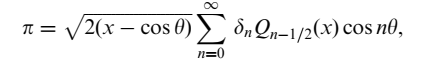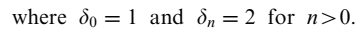Best

1.mw## How to draw a graph?...

Need help to draw a graph between x and lambda, lambda should on the horizontal axis. it should look like the attached picture.

 >>(1)
 >(2)
 >>(3)
 >Download help2.mw>>(1)
 >(2)
 >>(3)
 >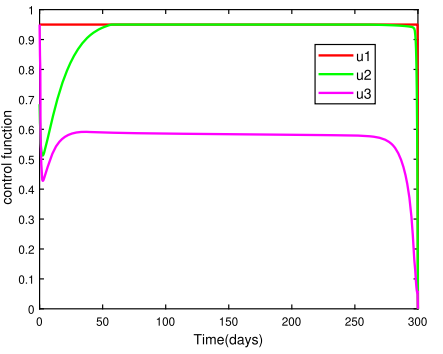1 2 3 4 5 6 7Last Page 1 of 1932